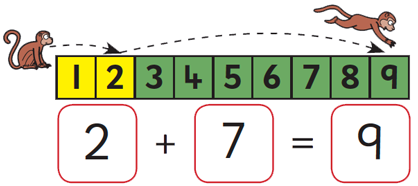Well done! That's the right answer.
HintTest
2
+
4
=
1
+
6
=
7
+
2
=
3
+
4
=
4
+
=
1
+
=
+
6
=
+
2
=
+
=
+
=
+
=
+
=
1
2
3
4
5
6
7
8
9